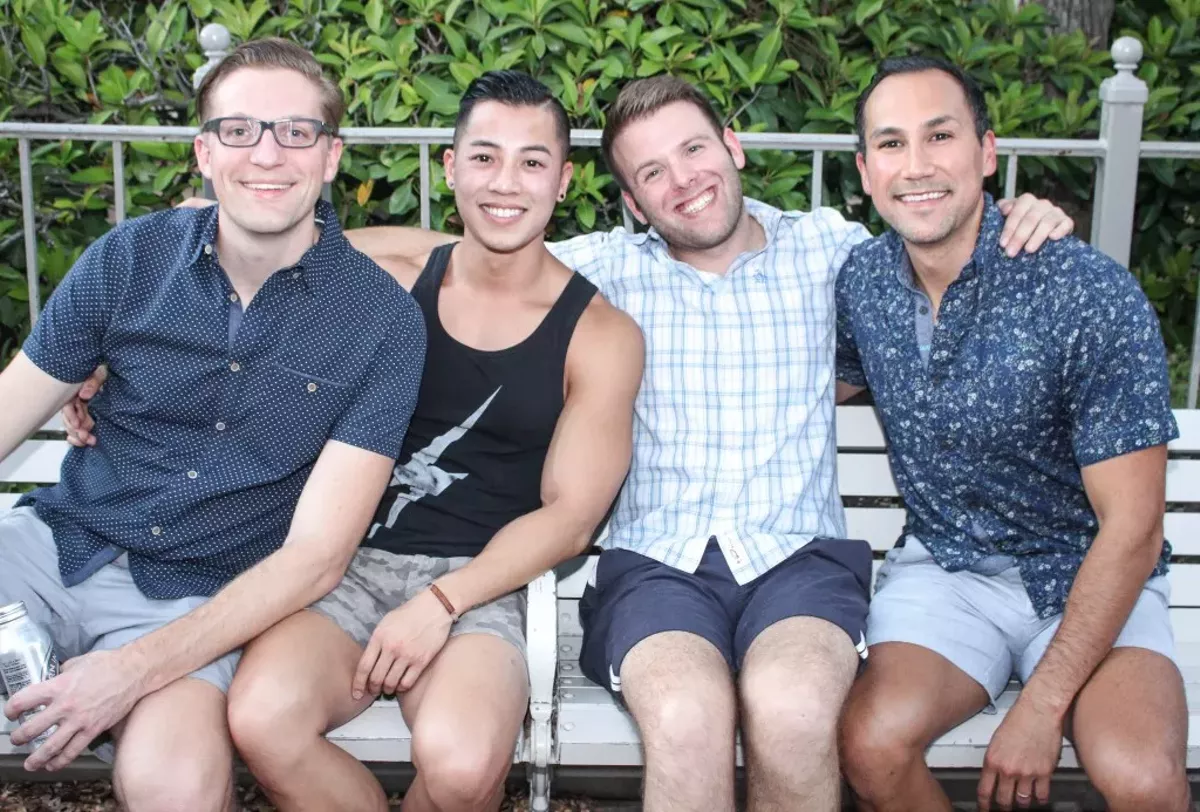# The Best Moments from Out in the Park 2016

Tweet
Submit to Reddit
Email
Presented by Rey Lopez Entertainment and held at Six Flags Fiesta Texas, Out in the Park is an LGBT private event that brings together roller coasters, rides and performances by 25 drag performers, including several from Ru Paul's Drag Race.
September 23, 2016
Photos by Julian P. Ledezma
OF 98
PREV NEXTJulian P. Ledezma
Julian P. Ledezma
Julian P. Ledezma
Julian P. Ledezma
Julian P. Ledezma
Julian P. Ledezma
Julian P. Ledezma
Julian P. Ledezma
Julian P. Ledezma
Julian P. Ledezma
Julian P. Ledezma
Julian P. Ledezma
Julian P. Ledezma
Julian P. Ledezma
Julian P. Ledezma
Julian P. Ledezma
Julian P. Ledezma
Julian P. Ledezma
Julian P. Ledezma
Julian P. Ledezma
Julian P. Ledezma
Julian P. Ledezma
Julian P. Ledezma
Julian P. Ledezma
Julian P. Ledezma
Julian P. Ledezma
Julian P. Ledezma
Julian P. Ledezma
Julian P. Ledezma
Julian P. Ledezma
Julian P. Ledezma
Julian P. Ledezma
Julian P. Ledezma
Julian P. Ledezma
Julian P. Ledezma
Julian P. Ledezma
Julian P. Ledezma
Julian P. Ledezma
Julian P. Ledezma
Julian P. Ledezma
Julian P. Ledezma
Julian P. Ledezma
Julian P. Ledezma
Julian P. Ledezma
Julian P. Ledezma
Julian P. Ledezma
Julian P. Ledezma
Julian P. Ledezma
Julian P. Ledezma
Julian P. Ledezma
Julian P. Ledezma
Julian P. Ledezma
Julian P. Ledezma
Julian P. Ledezma
Julian P. Ledezma
Julian P. Ledezma
Julian P. Ledezma
Julian P. Ledezma
Julian P. Ledezma
Julian P. Ledezma
Julian P. Ledezma
Julian P. Ledezma
Julian P. Ledezma
Julian P. Ledezma
Julian P. Ledezma
Julian P. Ledezma
Julian P. Ledezma
Julian P. Ledezma
Julian P. Ledezma
Julian P. Ledezma
Julian P. Ledezma
Julian P. Ledezma
Julian P. Ledezma
Julian P. Ledezma
Julian P. Ledezma
Julian P. Ledezma
Julian P. Ledezma
Julian P. Ledezma
Julian P. Ledezma
Julian P. Ledezma
Julian P. Ledezma
Julian P. Ledezma
Julian P. Ledezma
Julian P. Ledezma
Julian P. Ledezma
Julian P. Ledezma
Julian P. Ledezma
Julian P. Ledezma
Julian P. Ledezma
Julian P. Ledezma
Julian P. Ledezma
Julian P. Ledezma
Julian P. Ledezma
Julian P. Ledezma
Julian P. Ledezma
Julian P. Ledezma
Julian P. Ledezma
Julian P. Ledezma
1/98
Julian P. Ledezma

## Support Local Journalism

At a time when local-based reporting is critical, support from our readers is essential to our future. If you're able to, please support the San Antonio Current today.